Chapter 9 Force and Laws of Motion Class 9 Science Important Questions with Answers PDF will help you in scoring more marks in your exams.

## Force and Laws of Motion Class 9 Important Questions and Answers Science Chapter 9

Based on force and inertia

Question 1.
Name the physical quantity, which changes the shape of a ball.
Force.

More Resources

Question 2.
Define the term force. (CBSE 2011, 2012, 2014)
A force is a physical quantity which causes or tends to cause a motion in an object at rest or changes or tends to change the direction of motion of a moving object or the shape or size of the object.

Question 3.
Define balanced forces. (CBSE 2013)

Or

State the meaning of balanced forces. (CBSE 2012)
Two equal and opposite forces acting simultaneously on an object are known as balanced forces. The resultant force due to balanced forces is zero.

Question 4.
Define unbalanced forces. (CBSE 2013)
Two forces of unequal magnitudes and acting in opposite directions on an object simultaneously are known as unbalanced forces. The resultant force due to unbalanced forces is non zero.

Question 5.
Name the force which is responsible for change in position or state of an object. (CBSE 2012, 2013)
Unbalanced force.

Question 6.
A ball is moving over a horizontal smooth surface with a constant velocity. What type of forces are acting on the ball ?
Balanced forces are acting on the ball.

Question 7.
An unbalanced force is acting on an object. List two possible effects that will be caused in the motion of object.
(CBSE 2011)

1. Object may be accelerated or retarded,
2. Direction of motion of the object may change.

Question 8.
What do you mean by inertia ? (CBSE 2010, 2012)
The tendency of a body to oppose any change in its state of rest or uniform motion is called inertia of the body.

Question 9.
Write the kinds of inertia. (CBSE 2012)

1. Inertia of rest,
2. Inertia of motion
3. Inertia of direction.

Question 10.
Name the physical quantity that measures inertia. (CBSE 2010, 2012, 2015)
Mass of the body measures its inertia.

Question 11.
On which factor does inertia depend ? (CBSE 2013, 2015)
It depends on the mass of the object.

Question 12.
Which has more inertia : a man or a child ?
A man has more inertia than a child.

Question 13.
Which has highest inertia : solid made of aluminium, steel and wood oÇ same shape and same volume ?
(NCERT Question Bank, CBSE 2011, 2012)
Solid made of steel has the highest inertia because its mass is greater than aluminium and wooden solids.

Question 14.
Name the property of bodies to resist a change in their velocity. (CBSE 2012, 2013, 2014)
Inertia.

Question 15.
In a tug of war, the rope does not move in any direction. Why ? (CBSE 2013)
Because balanced forces act on the rope. Both the teams apply equal and opposite forces on the rope.

Question 16.
Which one has greater inertia : a stone of mass 1 kg or a stone of mass 5 kg ?
Inertia depends on the mass of the object. A stone of mass 5 kg has greater inertia than the stone of mass 1kg.

Question 17.
What kind of force is required to accelerate the motion of an object ?
Unbalanced force.

Question 18.
A ball is moving over a horizontal smooth surface with a constant velocity. What type of forces are acting on the ball ?
Balanced forces.

Question 19.
Name the forces (type of forces) when their resultant force acting on a body is not zero.
Unbalanced forces.

Question 20.
Mention any two affects of force.

1. It changes the state of rest or motion of a body,
2. It changes the shape of the body.

Question 21.
Is force a scalar or a vector quantity ?
Force is a vector quantity.

Question 22.
State Newtons first law of motion.
Or
Define the first law of motion.
Or
State the law of inertia.
Everybody continues in its state of rest or uniform motion in a straight line unless some external force acts on it.

Question 23.
Which law of motion defines the force ?
First law of motion.

Question 24.
Define momentum and give its SI unit. (CBSE 2010, 2011, 2012, 2014, 2015)
Momentum = mass x velocity.
SI unit of momentum is kg m s-1.

Question 25.
Name the factors on which momemtum of a body depends. (CBSE 2012, 2013, 2015)

1. Mass of the body and
2. Velocity of the body.

Question 26.
Define momentum of a body. (CBSE 2010, 2011)
Momentum of a body is defined as the product of the mass and velocity qf the body. Momentum of body = m x v.

Question 27.
Is momentum a scalar or a vector quantity ? (CBSE 2015)
Momentum is a vector quantity.

Question 28.
What is the name given to the product of mass and velocity of a body ? (CBSE 2012)
Linear momentum.

Question 29.
State Newtons second law of motion. Give its mathematical expression. (CBSE 2010, 2012, 2013)
The rate of change of momentum of a body is directly proportional to the unbalanced force acting on it and the change in momentum takes place in the direction of the unbalanced force. F = ma.

Question 30.
Name the physical quantity which is determined by the rate of change of linear momentum.
(CBSE 2010, 2012, 2013)
Force.

Question 31.
Define one unit of force. (CBSE 2010, 2011, 2013)
A force is said to be unit force if it produces unit acceleration in a body of unit mass.

Question 32.
Name the unit of force in SI.
newton (N).

Question 33.
Define SI unit of force. (CBSE 2011, 2014)
Or
Define one newton of force. (CBSE 2012)
SI unit of force is newton. Force is said to be 1 newton if it produces 1 m s-2 acceleration in a body of 1 kg.

Question 34.
What is the relationship between force and acceleration ? (CBSE 2012, 2013)
F = ma, where m is the mass of a body.

Question 35.
What is the name given to the product of mass and acceleration of a body ?
Force ( F = ma)

Question 36.
How much force acts on a body whose momentum is constant (i.e. p = constant) ?
F = dp/dt. If p = constant, then no force acts on the body.

Question 37.
Calculate the force required to produce an acceleration of 2.5 m s-2 in a body of 4 kg.
F = ma = 4 x 2.5 = 10 N.

Question 38.
A body of mass 30 kg has a momentum of 150 kg m s-1. Find its velocity. (CBSE 2013, 2014)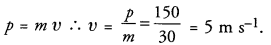Question 39.
An athlete always runs some distance before taking a jump. Why ?
To gain the momentum so that he may jump higher.

Question 40.
An object is thrown vertically upward. What is its momentum at the highest point ? (CBSE 2012)
Momentum = mass x velocity.
Since velocity of the object at the highest point is zero, so momentum of the object is zero at the highest point.

Question 41.
What will happen to the momentum of a body whose velocity is doubled ?
Momentum = mV. Therefore, momentum of a body is doubled if its velocity is doubled.

Question 42.
Name the physical quantity whose SI unit is “newton”.
Force.

Question 43.
Which would require a gereater force — accelerating a 1kg mass at 10 m s or a 2 kg mass at 4 m s ?
F = ma,
F1 = 1 x 10 = 10 N and
F2 = 2 x 4 = 8 N
So, 1 kg mass accelerating at 10 m s-2 requires greater force than the 2 kg mass at 4 m s-2.

Or

Which would require a greater force — accelerating a 2 kg mass at 5 m s-2 or a 5 kg mass at 3 ms-2 ?
F1 = m1 x a1 =2 x 5 = 10 N,
F2 = m2 x a2 = 5 x 3 = 15 N
F2 > F1

Question 44.
If the mass of a body is doubled, what will happen to its acceleration, if the applied force remains constant ?
a = F/m. If mass(m) is doubled, acceleration becomes half of its original value, if the applied force remains m constant.

Question 45.
State Newtons third law of motion.
To every action, there is equal and opposite reaction.

Question 46.
State action and reaction while a person walks on a ground.
Action : Force exerted by person on ground.
Reaction : Force exerted by ground on person.

Question 47.
Do action and reaction forces act on same body or different bodies ?
Action and reaction act on the different bodies.

Question 48.
State action and reaction in the case when a person swims.
Action : Force with which swimmer pushes the water in the backward direction with his hands.
Reaction : The force with which water pushes the swimmer in the forward direction.

Question 49.
State action and reaction in the case when a bullet is fired from the gun. (CBSE 2011)
Action : Force exerted by a spring on the bullet.
Reaction : Force exerted on the gun.

Question 50.
State action and reaction when a bullet is fired from the gun.
Force exerted on the bullet is action and the force exerted on the gun is reaction.

Question 51.
When a man jumps out from a boat to the bank of a river, the boat moves backwards. Identify the action and reaction in this situation. (CBSE 2015)
Action = force with which the foot of the man pushes the boat in the backward direction.
Reaction = force exerted by the boat on the man.

Based on the law of conservation of momentum

Question 52.
State law of conservation of momentum. (CBSE 2010, 2012, 2013, 2015)
The total momentum of a system remains constant if no external force acts on the system.

Question 53.
A fast moving truck loses momentum equal to 2 kg m s-1 after colliding with a stationary car.
Will the car lose or gain momentum ?
How much momentum is lost or gained by the car if the truck comes to the rest after collision ?
The car will gain momentum. Momentum gained by the car = 2 kg m s-1

Question 54.
Name the principle on which a rocket works. (CBSE 2012, 2013)
Law of conservation of linear momentum.

Question 55.
What do you mean by recoil velocity of a gun ?
The velocity with which a gun moves backward after firing a bullet is called the recoil of the gun.

Question 56.
Is momentum a scalar or a vector quantity ? (CBSE 2015)
Momentum is a vector quantity.

Based on force and inertia

Question 1.
When are the forces acting on a body said to be balanced ? Give an example. What type of change can the balanced forces bring about in an object ? (CBSE 2010, 2015)
Two forces of equal magnitude but acting in opposite directions on a body are said to be balanced forces. When we push a wall but the wall does not move at all, then balanced forces are acting on the wall. When balanced forces are -acting on an object, there is no change in the state of the object.

Question 2.
What are the changes that a force can bring about on a body?  Give examples. (CBSE 2010, 2011)

Or

Explain any three effects that can be produced by force with examples. (CBSE 2012, 2013, 2014)
Force can change

1. the shape of the body,
2. direction of motion .of the body,
3. the speed of the body and
4. size of the body

Examples :

1. The shape of a ball lying on a floor can be changed by pressing it.
2. The direction of motion of moving ball can be changed by hitting it with a bat.
3. A ball at rest can be set in motion if force is applied on it.
4. The length of a spring tied at one end can be increased by pulling it.

Question 3.
Two balls A and B of masses m and 2m are in motion with velocities ‘2V’ and ‘V’ respectively.
Compare
(i) their inertia,
(ii) their momentum and
(iii) the force needed to stop them in the same time.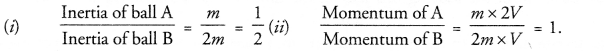(iii) Since momentum of both the balls is same, so same amount of force is needed to stop them in the same time.

Question 4.
Property of a body by virtue of which it opposes any change in state is called
(a) momentum
(b) energy
(c) inertia
(d) acceleration.
(c)

Question 5.
If A and B are two bodies with masses 10 kg and 50 kg respectively, then which body has more inertia ? Also calculate the ratio of the inertia of two bodies.
Inertia of body B is more than the inertia of body A.
body A 10 kg and body B 50 kg.
If the time taken to bring a ball to rest from a certain velocity V is reduced to half, what will be the change in values ofQuestion 6.
Look at the diagrams given (Figure A) and answer the following questions. In which case will the object move and in which direction ? Give reason in support of your answer.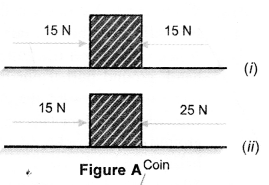In case (ii) object moves in the direction of force of 25 N because in this case the net force acting on the object = 25N-15N=10N
In case (i), net force acting on the object is zero.

Question 7.
In the given experimental set-up (Figure B) a student gave the card a sharp, fast horizontal flick with a finger.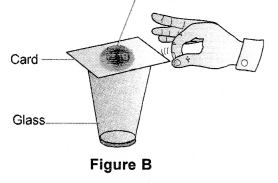1. What will happen to the coin ?

1. The coin will fall into the glass.
2. The card will move, when a fast horizontal flick with a finger is given to it. On the other hand, coin remains at rest due to inertia of rest and hence falls into the glass.

Question 8.
State the effect of force in each of the following cases,

1. A spring is stretched,
2. A hockey player hits an incoming ball
3. A football lying on the ground is kicked.

1. Length of spring increases and hence its shape is changed.
2. The direction of the ball is changed.
3. Football comes in motion from rest state.

Question 9.
(a) Initial and final momentum
(b) Change of momentum
(c) Rate of change of momentum.
(a) Initial and final momentum remain the same as they do not depend on time.
(b) Change in momentum remains the same as change in momentum = Final momentum-initial momentum.
(c)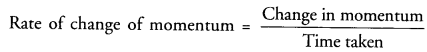therefore, rate of change of momentum is Time taken doubled if time is reduced to half.

Question 10.
While riding on the bicycle if we stop paddling, why does the bicycle begin to slow down ?
Bicycle begins to slow down as its motion is opposed by the unbalanced force known as force of friction between the types of bicycle and the road.

Based on Newton’s laws of motion

Question 11.
What happens when a wet piece of cloth is shaken ? (CBSE 2011)
When a wet piece of cloth is shaken, fibres of the cloth come in motion and water droplets in the cloth fall down due to inertia of rest.

Question 12.
A person getting down from the moving bus falls in the direction of the bus. Explain, why ?

Or

A passenger has to run along with moving bus in the same direction of the bus while getting down from the bus. Explain.

Or

It is is dangerous to jump out of a moving bus. Why ? (CBSE 2011, 2013)
As soon as the passenger gets down from a moving bus, his foot comes to rest but upper portion of his body remains in motion in the direction of the moving bus. He may fall down if he does not run along with the bus.

Question 13.
A stone tied to a string whirls in a horizontal circle. It flies off tangentially when the string breaks suddenly.
The stone flies off tangentially due to inertia of direction of motion.

Question 14.
What happens to a person travelling in a bus when the bus takes a sharp turn ? Explain.(CBSE 2011)

Or

A passenger in a moving car slips to one side of the seat when the car takes a sharp turn. Give reason for it. (CBSE 2012)
When car or bus takes a sharp turn, passenger slips to one side of the seat due to the inertia of motion.

Question 15.
It is difficult to balance our body when we accidendy step on a peel of banana. Explain, why ?(CBSE 2011)
When we walk on the ground, our foot pushes the ground in the backward direction (Action). On the other
hand, ground pushes our foot in the forward direction (Reaction). This reaction of the ground helps us to move in the forward direction.
But, when our foot falls on a peel of banana, then our foot slips on the peel of banana and cannot push the ground in the backward direction. Consequently, no reaction force acts on our foot. Hence we lose the balance and fall down.

Question 16.
Why are the wheels of vehicle provided with mud gaurds ? (CBSE 2012)
The rotating wheels of a vehicle throw out mud sticking to it tangentially due to inertia of direction. The mud
gaurds stop this mud to fall on another vehicle just moving behind the vehicle.

Question 17.
All the cars are provided with seat belts. Give reason. (CBSE 2012, 2013)
When a car stops suddenly, driver and passengers continue to move due to inertia of motion. Hence, they may
be hurt due to the collision between them and the body of the car. Therefore, seat belts are provided to prevent their forward motion in case of an accident.

Question 18.
A heavy leather ball and a light tennis ball are kept on the floor of a moving train. When train is suddenly stopped, they are set in motion. In which direction will they move ? Which one of them will attain higher velocity ?
They will move in the direction of the motion of the train due to inertia of motion. The mass, of leather ball is greater than that of tennis ball, so leather ball will resist more than the tennis ball to change its state of motion. Hence, tennis ball will attain higher velocity.

Question 19.
A bullet fired on a glass window makes a fine hole while a stone smashes when hits it. State the reason.
(CBSE 2015)
A bullet fired from a gun makes a small hole in the window pane while passing through it, but the stone striking the window pane breaks it into pieces. When a bullet strikes the window pane, only a small portion of the window pane where the bullet strikes comes in motion because the bullet makes contact with the pane for a very short time due to its high speed. On the other hand, the remaining portion of the window pane remains at rest due to inertia of rest. Thus, a small hole is made by the bullet in the window pane.
The speed of the stone is very small compared to the speed of the bullet. So the stone makes contact with the window pane for longer period of time. During this-longer time, the whole window pane comes in motion and hence breaks into pieces.

Question 20.
If the net external force acting on a body is zero, then total momentum of the body is
(a) Zero
(b) Variable
(c) Constant
(d) Information not sufficient.Question 21.
A body of mass ‘m is moving with a velocity u. When a force is applied on it for time t, its velocity increases to ‘v’. Write expressions for
(a) Initial and final momentum,
(b) Change in momentum
(c) Rate of change of momentum. Also write SI unit for each
(CBSE 2015)
(a) Initial momentum = mu
Final momentum = mv
(b) Change in momentum = final momentum – initial momentum = (mv – mu) = m(v – u)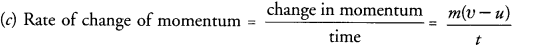SI unit of initial momentum, final momentum and change of momentum is kg m s-1
SI unit of rate of change of momentum is kg m s-2 or N.

Question 22.
If the time taken to bring a ball to rest from a certain velocity v is reduced to half, what will be the change in values of :
(a) Initial and final momentum
(b) Change of momentum
(c) Rate of change of momentum.
(a) Initial and final momentum remain the same as they do not depend on time.
(b) Change in momentum remains the same as change in momentum = Final momentum – initial momentum
(c)∴ Rare of change of momentum is doubled if time is reduced to half.

Question 23.
Derive First law of motion mathematically from the mathematical expression of Second law of motion.
According to First law of motion, a body continues to move with uniform velocity or zero acceleration if no external force acts on it. Second law of motion is given by F= ma.
If F = 0, a = 0. This is the mathematical expression of first law of motion.

Question 24.
Why, no force is required to move an object with a constant velocity ? (CBSE 2011)
We know, F = ma
When, velocity is constant, then acceleration, a = 0
Hence, F = 0

Question 25.
A cricket player lowers his hands while catching a fast moving ball. Explain. Why ?
(CBSE 2011, 2012, 2013)
A cricket player lowers his hands while catching the ball.
F = dp/dt
If a player does not lower his hands while catching the ball, the time to stop the ball is very small. So a large force has to be applied to reduce the velocity of the ball to zero or to change the momentum of the ball. When a player lowers his hands, the time to stop the ball is increased and hence less force has to be applied to cause the same change in the momentum of the ball. Therefore, the hands of the player are not injured.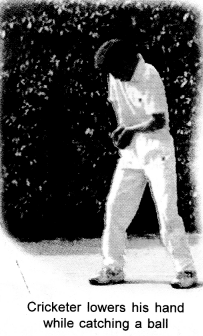Question 26.
Explain, how can we walk easily on a hard and rough surface than on a slippery surface ?
Walking of a person. When a person walks on the ground, he pushes the ground with his foot in the backward direction by pressing the ground. This push is known as action. According to Newtons third law of motion, an equal and opposite reaction acts on the foot of the person by the ground. This reaction (force) of the ground on the person pushes him forward (figure 21).
Thus, Action = force exerted on the ground by a person through his foot.Question 27.
Two similar buses are moving with same velocity on the road. One of them is empty and the other is loaded with passengers. Which of the two will be stopped by the application of less force ? Explain.
(CBSE 2010, 2011, 2013)
Momentum = m v. Loaded bus has greater mass than the empty bus. So loaded bus has greater momentum than the empty bus. The rate of change momentum is directly proportional to the force applied. Therefore, the empty bus will be stopped by applying less force.

Question 28.
A bullet fired from a gun is more dangerous than an air molecule hitting a person, though both bullet and air molecule are moving with same velocity. Explain.
The momentum of a bullet is very large than the momentum of the air molecule as the mass of bullet is very large than that of air molecule. When bullet and air molecule hit a person, then the momentum transferred to person by a bullet is very large than the momentum transferred by the air molecule. Hence, large force is exerted on the person by the bullet.

Question 29.
When a force acts on an object, it accelerates ? What do you observe, when two equal and opposite forces act on an object ? (CBSE 2011)
When two equal and opposite forces act on an object, then the net force acting on it is zero. Therefore, the object will remain at rest if it were at rest before the application of two equal and opposite forces. However, the object will continue to move with constant velocity if it were moving with constant velocity before the application of the forces.

Question 30.
Draw velocity-time graph of a moving particle on which net external force acting is zero.
Net external force = zero. It means, acceleration (a = F/m) of the particle is zero.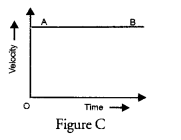In other words, particle is moving with constant velocity. Velocity-time graph of such a particle is represented by a curve AB parallel to time-axis (Figure C)

Question 31.
Draw distance-time graph of a moving object on which net external force acting is zero.
Net external force = zero. It means, acceleration (a = F/m) of the particle is zero.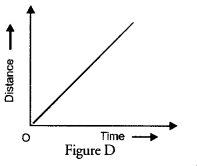In other words, the object is moving with constant velocity. Therefore, the particle travels equal distances in equal intervals of time. Distance time graph of such an object is shown in figure D.

Question 32.
Glass wares are wrapped in straw during their transportation. Give reason.
(CBSE 2012)
During transportation, the glass wares may break if they collide with each other in the event of jerks. When they are wrapped in straw, the force of jerk is transmitted to them through the pieces of straw in a longer period of time. Thus, the change in momentum of the glass wares takes place in a longer period of time. Therefore, a very small force is experienced by them in the event of jerks and hence they do not break.

Question 33.
When two bodies X and Y collide with each other, X exerts a force of 5 N on Y towards east direction. What
is the force exerted by Y on X ? Justify your answer stating the law. (CBSE 2012)
Force exterted by Y on X = 5 N towards west direction as per Newton’s third law of motion.

Question 34.
Explain, how a Karate player can break a pile of tiles with a single blow of his hand. (CBSE 2012)
A Karate player strikes the pile of tiles very fast so that change in the momentum of his hand takes place in
very small time interval. Hence, a very large force (F = dp/dt) is exerted on the pile of tiles, which is enough to break the pile of tiles.

Question 35.
Why a glass pane of a window is shattered when a flying pebble hits it ? (CBSE 2012)
When a flying pebble hits the glass pane, its contact time with glass pane is large. During this large interval of time, it imparts its momentum to the entire glass pane, which in turn shattered.

Question 36.
A truck of mass M is moved under a force F. If the truck is loaded with an object equal to the mass of the truck and the driving force is halved, then how does the acceleration change ?
Acceleration, a = F/m. When m is doubled and F is made half, acceleration becomes ¼ th of its initial value.

Question 37.
Why are road accidents at high speed very much worsen than accidents at low speeds ?
When accidents at high speed occur, then large momentum is to be changed in small interval of time. Hence, large force is exerted on vehicles and they get damaged very badly as compared to when accidents occur at low speed.

Question 38.
Name the physical quanity whose unit is

1. kg m s-2 and
2. kg m s-1

1. Force,
2. Linear momentum

Question 39.
Represent the following graphically :
(a) momentum versus mass, when velocity is constant
(b) momentum versus velocity, when mass is constant.
(a)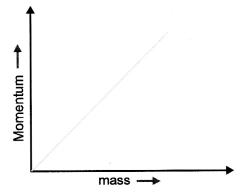(b)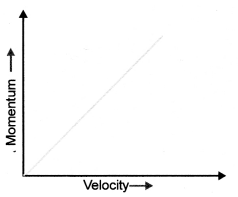Question 40.
Describe in brief an activity to illustrate the properly of inertia of rest.

1. Describe an activity to demonstrate the property of inertia of rest of an object.
2. Now place a fifty paisa coin on the card board.
3. Strike the card board with a finger.

It is found that card board flies away and the coin falls into the glass. This is because, card board moves away due to the application of force of finger. However, coin remains at its position due to inertia of rest and hence falls into the glass.
This activity demonstrates the property of inertia of rest of an object. It is based on Newton’s first law of motion.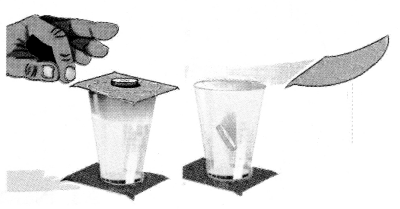Question 41.
In the figure below, the card is flicked with a push.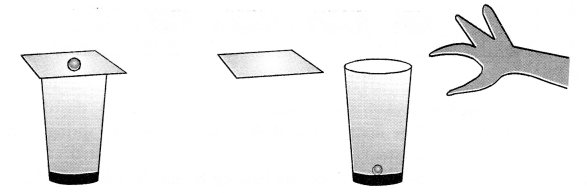(a) What do you observe in above case and why ?
(b) State the law involved in this case.
(c) What will be your observation if the above coin is replaced by a heavy five rupee coin. Justify your answer.
(a) The coin falls into the glass. The card comes in motion due to push but coin on the card remains at rest. The coin falls into the glass due to inertia of rest.
(b) Law of inertia or First law of motion.
(c) Heavy coin will also fall into the glass. The heavy coin has more inertia than the lighter coin.

Question 42.
State Newton’s Third law of motion. Are the forces mentioned in the law balanced forces ? Why ? Will they always produce accelerations of equal magnitude ? Why ?
For every action, there is equal and opposite reaction. Forces are not balanced forces because both the forces act on two different bodies. They do not produce the same acceleration because acceleration = ~~. Since masses (m) of both the bodies are different, so accelerations of both the bodies are different.

Question 43.
When a person hits a stone, his foot is injured. Why ? (CBSE 2011)
When a person hits a stone, the stone exerts equal force on his foot. Due to this force, his foot gets injured.

Question 44.
According to Newton’s third law of motion, a ball falling towards earth exerts a force on the earth but the motion of earth towards the ball is not noticed. Explain why ?
The acceleration produced in the earth is very small as its mass is very large. Hence, the motion of the earth towards ball is not noticed.

Question 45.
How a man can swim in water ?

Or

A swimmer is able to swim in a forward direction in a swimming pool only when he is pushing the water in the backward direction. Give reason for the above mentioned statement and justify the same. (CBSE 2013)
When a swimmer pushes water in the backward direction with his hands, equal and opposite reaction by water on the man pushes him in the forward direction.

Question 46.
When a person jumps out of a boat, the boat moves backward. Explain, why ? (CBSE 2011, 2012)
When a person jumps out of a boat, he pushes the boat in the backward direction (Action). According to Newtons third law of motion, the boat exerts an equal force (i.e. reaction) on the person in the forward direction. So, when the person jumps out of the boat, the boat moves backward (Figure 23). Thus, Action = force applied by the person on the boat through his foot. Reaction = force exerted on the person by the boat.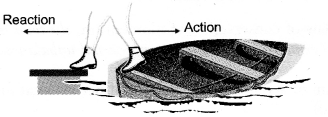Question 47.
Why a boatman pushes the bank of the river with a pole to take his boat into the river ?
A boatman pushes the river bank with a bamboo pole to take his boat into the river.
When the boatman pushes the river bank with a bamboo pole, the river bank offers an equal and opposite reaction. This reaction helps the boat to move into the river. In this case, action = force applied on the ground with a bamboo pole, and reaction = force exerted by the ground on the boat.Question 48.
“Action and reaction are equal and opposite but even then they do not cancel each other.” Explain, why ?
(CBSE 2011)

Or

Why don’t, the forces of action and reaction cancel each other ? (CBSE 2013)
Two equal and opposite forces can cancel each other if they act on the same body. But action and reaction do not act on the same body. Action acts on one body and the reaction acts on another body. Hence they cannot cancel each other.

Question 49.
Look at the diagram and answer the following questions :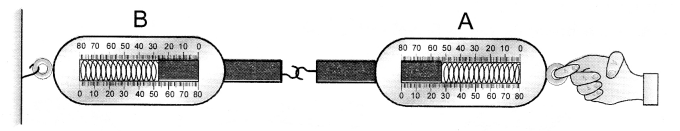(a) When a force is applied through the free end of the spring balance A, the reading on the spring balance A is 15 g wt. What will be the measure of the reading shown by spring balance B ?
(c) Name the force which balance A exerts on balance B and the force of balance B on balance A.
(a) Reading shown by spring balance is 15 g wt.
(b) Every action has equal and opposite reaction.
(c) Force which balance A exerts on balance B is action. Force of balance B on balance A is reaction.

Question 50.
A man throws a ball weighing 500 g vertically upwards with a speed of 10 m s-1
(i) Find its initial momentum
(ii) Find its momentum at the highest point of its flight.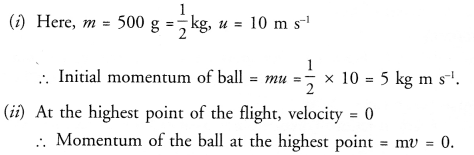Question 51.
A person is hit harder, when he falls on a hard floor than when he falls on the heap of sand or cotton. State the reason. (CBSE 2015)
According to Newton’s second law of motion,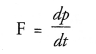When a person falls on a hard floor, his change in momentum occurs in small interval of time because he comes to rest abruptly. Hence, a large force is exerted on the person by the floor. On the other hand, when the person falls on a heap of sand or cotton, the sand or cotton yields under his weight. Therefore, the change in momentum of the person occurs in a large interval of time. Hence, less force is exerted on the person by the heap of sand or cotton.

Question 52.
Mention any two examples which can be explained on the basis of Third law of motion.

1. Walking of a person on a road,
2. Recoil of a gun.

Based on the law of conservation of momentum

Question 53.
Why does a gun recoil backward when a bullet is fired from it ?

Or

Firing a bullet from a gun, results in recoil of gun. Give reason. (CBSE 2011)
A gun recoils, when a bullet is fired from it to conserve the linear momentum of the gun and the bullet.

Question 54.
A gun recoils backward with small speed than the bullet moving forward. Explain why ?
Recoil speed of gun is inversely proportional to the mass of the gun. Mass of gun is much greater than the mass of bullet. Therefore, gun recoils backward with small speed than the bullet moving forward.

Question 55.
Explain, why an inflated balloon lying on the surface of a floor moves forward when pierced with a needle.
When balloon is pierced with a needle, air inside the balloon rushes out with certain momentum. Therefore, the balloon moves in the opposite direction to conserve the linear momentum of the balloon.

Question 56.
When a bullet is fired, it exerts an equal and opposite force on the gun, yet hurt caused by the recoil of the
gun is much less than that by a bullet ? Give reason. (CBSE 2012)
Recoil velocity of gun is much less than the velocity of bullet. Therefore, momentum imparted by gun is very small and hence it hurts less than that of the bullet.

Question 57.
Mention two examples from your daily life which can be explained on the basis of law of conservation of momentum. (CBSE 2013)

1. Recoil of a gun,
2. Rocket propulsion.

Question 58.
Two friends on roller skates are standing 5m apart facing each other. One of them throws a ball of 2 kg towards the other, who catches it ? How will this activity affect the positions of the two ? Explain.
Total initial linear momentum of the two friends is zero as both are at rest. When one person throws the ball towards the other and the other catches it, then the total linear momentum of both will have to be zero as no external force acts on them. Thus both friends must move in opposite direction. Hence, the distance between both friends will increase.

Question 59.
Observe the following diagram and answer the questions given below :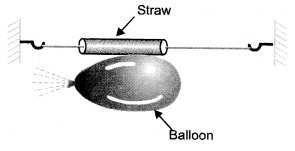Which direction does the balloon move when the thread tied to its neck is removed and why ?
State the conclusion drawn from this activity.
When the thread tied to its neck is removed, air inside the balloon rushes to the left side and the balloon moves to right side to conserve linear momentum.
Law of conservation of linear momentum is verified.

Question 60.
A gunman gets jerk in backward direction while firing a gun. State the reason. (CBSE 2015)
The gun recoils backward to conserve the momentum of the system (gun + bullet). The backward motion of the gun exerts a force on the gunman and hence he gets a jerk in the backward direction.

Question 1.
State Newtons second law of motion. Use this law to find the method to measure force acting on an object.
(CBSE 2010, 2011)

Or

From Newton’s second law of motion, obtain a mathematical expression for force. (CBSE 2013)
According to this law, the change in momentum of a body per unit time (i.e. rate of change of momentum) is directly proportional to the unbalanced force acting on the body and the change in momentum takes place in the direction of the unbalanced force on the body.
F ∞ dp/dt
Consider a body of mass moving with initial velocity Let a force acts on the body for time so that the velocity of the body after time is .
Initial momentum of the body, pi = mu
Final momentum of the body, pf = mv
Now, change in momentum of the body = pf – p= mv – mu = m(v-u)Thus, force acting on the body is directly proportional to (i) its mass (m) and (ii) its acceleration (a).
Eqn. (1) gives the mathematical form of Newtons second law of motion. The force given by eqn (1) acts on the body.
Newton’s second law of motion in vector form
We know, force ( F ) and acceleration ( a ) are vector quantities, whereas mass ( m)  is a scalar quantity. Therefore, Newton’s second law of motion can be written in vector form asThis relation shows that the direction of force applied on the body is same as that of the acceleration produced in the body.

Question 2.
Define momentum of a body. Prove with the help of third law of motion that the total momentum of two bodies is conserved during collision provided no external force acts. (CBSE Sample Paper)
According to the law of conservation of momentum, the total momentum of a system (or an object) remains constant if no net external unbalanced force acts on the system.
That is, momentum of a system, p = constant, if net external force acting on it is zero (i.e. F = 0).
Consider a system consisting of two bodies A and B of (say glass spheres) masses m1 and m2 respectively. Let these bodies are moving with velocities uand u2 as shown in figure 26. Let u1 > u2 Let these bodies collide with each other for a small interval of time ‘dt’. At the time of collision, body A exerts a force F on body B and body B exerts equal and opposite force (- F) on body A. Due to these forces, the momentum of the bodies changes. Let v1 and v2 be the velocities of body A and body B respectively after the collision.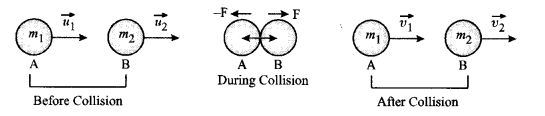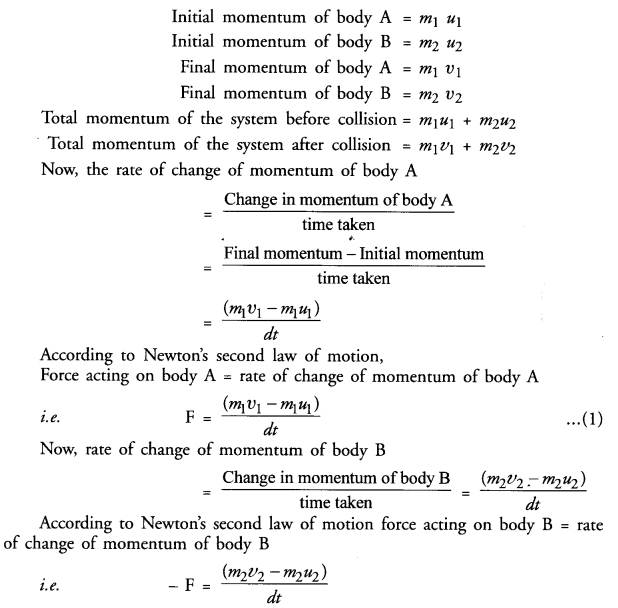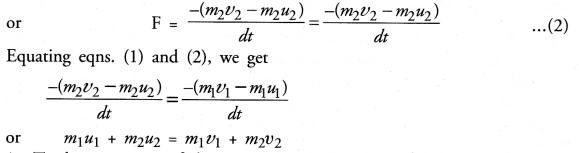i.e. Total momentum of the system (i.e. bodies A and B) before collision = Total momentum of the system (i.e. bodies A and B) after collision. This is the law of conservation of linear momentum.

Question 3.
From Newton’s second law of motion,From this establish F = ma, where m is mass and a is acceleration. (CBSE 2011)
Consider a body of mass moving with initial velocity Let a force acts on the body for time so that the velocity of the body after time is .
Initial momentum of the body, pi = mu
Final momentum of the body, pf = mv
Now, change in momentum of the body = pf — p= mv – mu = m(v-u)Thus, force acting on the body is directly proportional to (i) its mass (m) and (ii) its acceleration (a).
Eqn. (1) gives the mathematical form of Newtons second law of motion. The force given by eqn (1) acts on the body.
Newton’s second law of motion in vector form
We know, force ( F ) and acceleration ( a ) are vector quantities, whereas mass ( m)  is a scalar quantity. Therefore, Newton’s second law of motion can be written in vector form as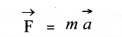This relation shows that the direction of force applied on the body is same as that of the acceleration produced in the body.

Question 4.
Derive the law of conservation of linear momentum.

Or

Total momentum of two bodies remains unchanged before and after the collision. Justify this statement.
(CBSE 2015)
Let a moving ball collides with another stationary ball lying on a ground. Observe, what happens after collision. The moving ball will slow down i.e. its velocity decreases after colliding with the stationary ball. On the other hand, the stationary ball begins to move i.e. its velocity increases after collision.
Consider a system consisting of two bodies A and B of (say glass spheres) masses m1 and m2 respectively. Let these bodies are moving with velocities uand u2 as shown in figure 26. Let u1 > u2 Let these bodies collide with each other for a small interval of time ‘dt’. At the time of collision, body A exerts a force F on body B and body B exerts equal and opposite force (- F) on body A. Due to these forces, the momentum of the bodies changes. Let v1 and v2 be the velocities of body A and body B respectively after the collision.i.e. Total momentum of the system (i.e. bodies A and B) before collision = Total momentum of the system (i.e. bodies A and B) after collision. This is the law of conservation of linear momentum.

Question 5.
State and verify law of conservation of momentum. (CBSE 2013)